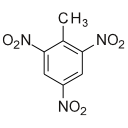Subsections

## structure.superimpose### Synopsis

Superimpose a set of structures.

### Defaults

structure.superimpose(pipes=None, models=None, molecules=None, atom_id=None, displace_id=None, method=`fit to mean', centre_type=`centroid', centroid=None)

### Keyword arguments

pipes: The data pipes to use in the superimposition.

models: The list of lists of models for each data pipe to use in the superimposition. The number of elements in the first dimension must match the pipes argument. If no models are given, then all will be used.

molecules: The list of molecules for each data pipe to use in the superimposition. This allows differently named molecules in the same or different data pipes to be superimposed. The number of elements must match the pipes argument. If no molecules are given, then all will be used.

atom_id: The atom identification string of the coordinates of interest. This allows a subset of all residues or atoms to be used in the superimposition. For example for protein backbone heavy atoms, this can be set to `@N,C,CA,O'.

displace_id: The atom identification string for restricting the displacement to a subset of all atoms. If not set, then all atoms will be translated and rotated. If supplied as a list of IDs, then the number of items must match the number of structures.

method: The superimposition method.

centre_type: The type of centre to user for the superimposition, i.e. either the standard centroid superimposition or a superimposition using the centre of mass (CoM).

centroid: The alternative position of the centroid.

### Description

This allows a set of related structures to be superimposed to each other. If a multiple sequence alignment (MSA) of the molecules has already been performed with the structure.sequence_alignment user function, this will allow residues with different numbering to be superimposed. Otherwise only residues with the same numbering will be used in the superimposition. Two superimposition methods are currently supported:

`fit to mean' -
All models are fit to the mean structure. This is the default and most accurate method for an ensemble description. It is an iterative method which first calculates a mean structure and then fits each model to the mean structure using the Kabsch algorithm. This is repeated until convergence.
`fit to first' -
This is quicker but is not as accurate for an ensemble description. The Kabsch algorithm is used to rotate and translate each model to be superimposed onto the first model of the first data pipe.

Support for multiple structures is provided by the data pipes, model numbers and molecule names arguments. Each data pipe, model and molecule combination will be treated as a separate structure. As only atomic coordinates with the same residue name and number and atom name will be assembled, structures with slightly different atomic structures can be compared. If the list of models is not supplied, then all models of all data pipes will be used. If the optional molecules list is supplied, each molecule in the list will be considered as a separate structure for comparison between each other.

The atom ID string, which uses the same notation as the spin ID, can be used to restrict the coordinates compared to a subset of molecules, residues, or atoms. For example to only use backbone heavy atoms in a protein, set the atom ID to `@N,C,CA,O', assuming those are the names of the atoms in the 3D structural file.

The displacement ID string, which is similar to the atom ID, gives finer control over which atoms are translated and rotated by the algorithm. When not set this allows, for example, to align structures based on a set of backbone heavy atoms and the backbone protons and side-chains are displaced by default. Or if set to the same as the atom ID, if a single domain is aligned, then just that domain will be displaced.

By supplying the position of the centroid, an alternative position than the standard rigid body centre is used as the focal point of the superimposition. The allows, for example, the superimposition about a pivot point.

### Prompt examples

To superimpose all sets of models, type one of:

```[numbers=none]
relax> structure.superimpose()
```

```[numbers=none]
relax> structure.superimpose(method='fit to mean')
```

To superimpose the models 1, 2, 3, 5 onto model 4, type:

```[numbers=none]
relax> structure.superimpose(models=[[4, 1, 2, 3, 5]], method='fit to first')
```

To superimpose an ensemble of protein structures using only the backbone heavy atoms, type one of:

```[numbers=none]
relax> structure.superimpose(atom_id='@N,C,CA,O')
```

```[numbers=none]
relax> structure.superimpose(method='fit to mean', atom_id='@N,C,CA,O')
```

To superimpose the structures in the `A' data pipe onto the structures of the `B' data pipe using backbone heavy atoms, type one of:

```[numbers=none]
relax> structure.superimpose(['B', 'A'], None, 'fit to first', '@N,C,CA,O')
```

```[numbers=none]
relax> structure.superimpose(pipes=['B', 'A'], method='fit to first', atom_id='@N,C,CA,O')
```

The relax user manual (PDF), created 2020-08-26.